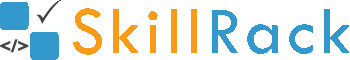How to get a space separated String as input in C1 year ago

Accepting space separated String value in C Programming Language:

In C, using %s in scanf accepts a string value only till the first non-white space character. For example, the below program will print only One when One Two Three is given as input.

#include <stdio.h>

int main()
{
char inputStringValue;
scanf("%s",inputStringValue);
printf("%s",inputStringValue);
}

So to accept a string with space we can use the following approaches.

Approach 1:
Use fgets to accept the input string value (which has one or more spaces in it).
This program will print One Two Three when One Two Three is given as input.

#include <stdio.h>

int main()
{
char inputStringValue;
fgets(inputStringValue,100,stdin);
printf("%s",inputStringValue);
}


Approach 2:
Use ^\n to instruct the scanf to read till a new line character is encountered in the input value.

#include <stdio.h>

int main()
{
char inputStringValue;
scanf("%[^\n]",inputStringValue);
printf("%s",inputStringValue);
}